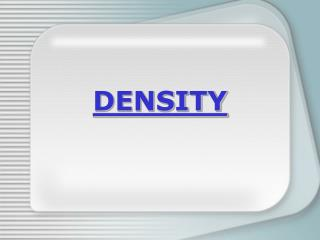DownloadDownload PresentationDENSITY

# DENSITY

Télécharger la présentation## DENSITY

- - - - - - - - - - - - - - - - - - - - - - - - - - - E N D - - - - - - - - - - - - - - - - - - - - - - - - - - -
##### Presentation Transcript

1. DENSITY

2. What IS density? • Science Definition: Mass per unit volume • Every day Definition: How heavy something is for its size.

3. Let’s Review: MASS and VOLUME • Mass: • how much matter is in an object, or how “heavy” something is. • Volume: • how much space something takes up.

4. 10 cm 5 cm 10 cm How do you measure mass and volume? • Mass: • use a scale • Volume: 3 Ways to measure • use a graduated cylinder • measure the length, width, and height of something • see how much water it displaces.

5. How do we calculate Density? • Density = Mass/Volume • Units of mass = ? • grams • Units of Volume = ? • liter (we will use mL or cm3 in class REMEMBER 1mL = 1cm3) • Units of Density = ? • grams/cm3

6. A B What determines density? • Tube example--which is more dense? • The one with more marbles • WHY? • There is more mass in the tube that has more marbles • If you pack more mass into the same volume, it’s more dense.

7. A B What determines density? • Foam example--which is more dense? • The one that is more squished. • WHY? • Because it’s packed into a smaller volume. • If you pack the same mass into a smaller volume, it’s more dense.

8. What determines density? • Styrofoam and paper clip example--which is more dense? • The paper clip. • WHY? • Because the paper clip (metal) is heavier for its size. • Just because something has more mass doesn’t mean it’s more dense.

9. Population Density

10. Making Connections • Is your overall density (the density of your body) the same as or different from the density of water? If it is not the same, is it more or less? How do you know?

11. Making Connections • Think of a toy that is designed to have a density that is greater than water. Why is it designed that way?

12. Making Connections • Think of a toy that is designed to have a density that is less than water. Why is it designed that way?

13. Making Connections • How do submarines use relative density in order to work? • What would happen if a fish always had a density less than water or greater than water?

14. X Calculating Density • d = m/v • m = d x v • v = m/d • Make a triangle like this in your notes: Mass M Density D Volume V

15. Calculating Density • Bob has 50 grams of water in a cup. When he pours it into a graduated cylinder, he finds that the volume of that water is 50 mL. What is the density of water? • What is known? • Mass = 50 grams • Volume = 50 mL • What do we do with these numbers? • D = m/v • D = 50 g/50 mL • D = 1g/mL (OR 1g/cm3)

16. What happens to density if we… • What if we have BIG mass and small volume? • Ex: BIG MASS = 500 g • Small volume = 10 cm3 • Density = ? • 500g / 10cm3 • 50 g/cm3 • Would it sink or float? • Sink • How do you know? • The density of water is 1g/cm3, so it’s more dense than water = sink

17. What happens to density if we… • What if we have small mass and BIG volume? • Ex: small mass = 5 g • BIG volume = 100 cm3 • Density = ? • 5g / 100cm3 • 0.05 g/cm3 • Would it sink or float? • Float • How do you know? • The density of water is 1g/cm3, so it’s less dense than water = float

18. How do these work? • Galileo thermometer • Tube of gravel, sand, clay and silt. • Lava Lamp

19. Pop Can Challenge • What do you think will happen when I place a can of diet cola in water? • What do you think will happen when I place a can of regular cola in water? • How about lemon lime soda? • How does the density of something that sinks compare to the density of the fluid surrounding it? • How does the density of something that floats compare to the density of the fluid surrounding it? • What causes an object to remain sunk? • What causes and object to remain afloat? • What would happen if we added corn syrup to the water? WHY?

20. Your task today… • You need to figure out the density of these six different cubes. • You and your group members (2-3 people) are in charge of measuring the density of each cube. • What you will need to write down and turn in (one per person) • Procedure--step by step how to calculate density (be detailed so others could follow!) • A data table of the data you collect • A list of the density (with proper units) of each cube A - F.